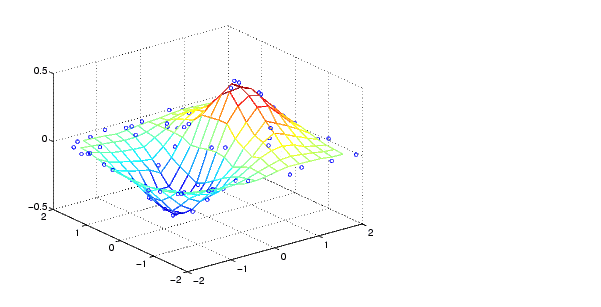MATLAB Function Referencegriddata

Data gridding

Syntax

• ```ZI = griddata(x,y,z,XI,YI)
[XI,YI,ZI] = griddata(x,y,z,XI,YI)
[...] = griddata(...,method)
```[...] = griddata(...,method,options)
``````

Description

```ZI = griddata(x,y,z,XI,YI) ``` fits a surface of the form `z = f(x,y)` to the data in the (usually) nonuniformly spaced vectors `(x,y,z)`. `griddata` interpolates this surface at the points specified by `(XI,YI)` to produce `ZI`. The surface always passes through the data points. `XI` and `YI` usually form a uniform grid (as produced by `meshgrid`).

`XI` can be a row vector, in which case it specifies a matrix with constant columns. Similarly, `YI` can be a column vector, and it specifies a matrix with constant rows.

```[XI,YI,ZI] = griddata(x,y,z,XI,YI) ``` returns the interpolated matrix `ZI` as above, and also returns the matrices `XI` and `YI` formed from row vector `XI` and column vector `yi`. These latter are the same as the matrices returned by `meshgrid`.

```[...] = griddata(...,method) ``` uses the specified interpolation method:

 `'linear' ` Triangle-based linear interpolation (default) `'cubic'` Triangle-based cubic interpolation `'nearest'` Nearest neighbor interpolation `'v4'` MATLAB 4 `griddata` method

The `method` defines the type of surface fit to the data. The `'cubic'` and `'v4'` methods produce smooth surfaces while `'linear'` and `'nearest'` have discontinuities in the first and zero'th derivatives, respectively. All the methods except `'v4'` are based on a Delaunay triangulation of the data. If `method` is `[]`, then the default `'linear'` method is used.

`[...] = griddata(...,method,options)` specifies a cell array of strings `options` to be used in Qhull via `delaunayn`. If `options` is `[]`, the default `delaunayn` options are used. If `options` is `{''}`, no options are used, not even the default.

Occasionally, `griddata` might return points on or very near the convex hull of the data as `NaN`s. This is because roundoff in the computations sometimes makes it difficult to determine if a point near the boundary is in the convex hull.

Remarks

`XI` and `YI` can be matrices, in which case `griddata` returns the values for the corresponding points `(XI(i,j),YI(i,j))`. Alternatively, you can pass in the row and column vectors `xi` and `yi`, respectively. In this case, `griddata` interprets these vectors as if they were matrices produced by the command `meshgrid(xi,yi)`.

Algorithm

The `griddata(...,'v4')` command uses the method documented in . The other `griddata` methods are based on a Delaunay triangulation of the data that uses Qhull . For information about Qhull, see http://www.qhull.org/. For copyright information, see http://www.qhull.org/COPYING.html.

Examples

Sample a function at 100 random points between ±`2.0`:

• ```rand('seed',0)
x = rand(100,1)*4-2; y = rand(100,1)*4-2;
z = x.*exp(-x.^2-y.^2);
```

`x`, `y`, and `z` are now vectors containing nonuniformly sampled data. Define a regular grid, and grid the data to it:

• ````ti` `=` `-2:.25:2;`
`[XI,YI]` `=` ```meshgrid(ti,ti);
````ZI` `=` ```griddata(x,y,z,XI,YI);
``````

Plot the gridded data along with the nonuniform data points used to generate it:

• ``````mesh(XI,YI,ZI), hold
````plot3(x,y,z,'o'), hold` ```off
``````

See Also

`delaunay`, `griddata3`, `griddatan`, `interp2`, `meshgrid`

References

  Barber, C. B., D.P. Dobkin, and H.T. Huhdanpaa, "The Quickhull Algorithm for Convex Hulls," ACM Transactions on Mathematical Software, Vol. 22, No. 4, Dec. 1996, p. 469-483. Available in PDF format at http://www.acm.org/pubs/citations/journals/toms/1996-22-4/p469-barber.

  National Science and Technology Research Center for Computation and Visualization of Geometric Structures (The Geometry Center), University of Minnesota. 1993.

  Sandwell, David T., "Biharmonic Spline Interpolation of GEOS-3 and SEASAT Altimeter Data", Geophysical Research Letters, 2, 139-142,1987.

  Watson, David E., Contouring: A Guide to the Analysis and Display of Spatial Data, Tarrytown, NY: Pergamon (Elsevier Science, Inc.): 1992.

© 1994-2005 The MathWorks, Inc.